## Audio

[Audio][slideshow]

## Frequency to Voltage converter circuit based on TC9400 IC.

Description.
A very simple and low cost frequency to voltage converter based on the TC9400 IC from Microchip is shown here. TC9400 can be either wired as a voltage to frequency converter or frequency to voltage converter and it requires minimum external components. The functional blocks inside the TC9400 includes integrator opamp, 3uS delay circuit, one shot circuit, charge discharge control circuit, divide by 2 network and necessary drivers. This circuit finds application in a range of electronic projects like frequency meters, tachometers, speedometers, FM demodulators etc.

Circuit diagram.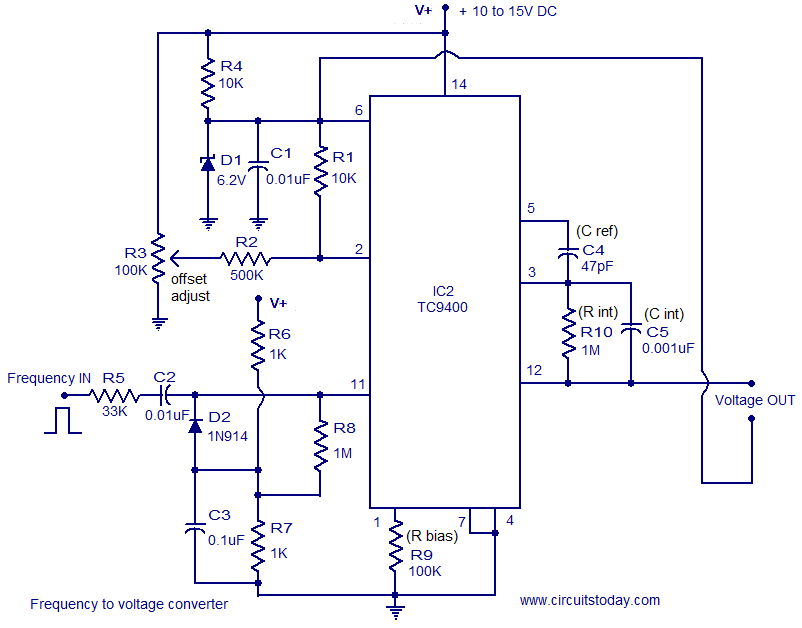TC9400 Voltage to Frequency converter (Single supply version)

In the circuit shown above the TC9400 is wired as a F to V converter that operates from a single supply. The circuit generates an output voltage that is proportional to the input frequency. The input frequency is applied to the pin11 (non inverting input of the internal comparator). In order to trip the comparator the amplitude of the input frequency must be greater than +/-200mV.Below this level the circuit will not work at any situation.

Whenever the input signal to the pin 11 of IC1 crosses zero to the negative direction the output of the internal comparator goes low. The 3uS delay circuit enables the Cref charge/discharge circuit after 3uS and this connects the Cref to the reference voltage and this charges the integrating capacitor Cint a specific amount of voltage. In the single supply operation the reference voltage is the potential difference between pin 2 and 7 of the TC9400. Each time the input frequency wave form crosses zero towards positive direction, the output of the internal comparator goes high and this disables the Cref charge/discharge circuit which creates a short circuit across the Cref leads. The voltage across the integrating capacitor Cint is retained because the only discharge path available is the 1M resistor Rint which is a too high and the voltage across Cint is the output voltage. Resistor Rbiasis used to set the bias current of the IC.

The potential divider network comprising of R6 and R7 makes sure that the input threshold tracks the supply voltage always. The clamp circuit using diode D2 prevents the input from going far negative in order to turn on the internal comparator. In simple words this section of the circuit can be generally termed as a level shifter.

The TC9400 manufacturers claim that it can accept a signal of any frequency at its input. In the practical side, for the proper working of this circuit the positive half of the input signal must have at least 5uS pulse width and for the negative half it must be greater than or equal to 5uS.

For calibration adjust the offset adjust trimpot to obtain 0V at the output with no input frequency applied. If you have a function generator, set frequency input to 10KHz and make adjustments in the value of Cref to get around 2.5 to 3 volts at the output. This calibration is meant for a maximum input frequency of 10KHz.

Notes.

• The circuit can be assembled on a Perf board or PCB.
• The circuit can be powered from anything between 10 to 15V DC.
• R3 can be used for adjusting the offset voltage.
• In the circuit the inverting input of the internal comparator is referenced to 6.2V by using D1. So the input signals amplitude must be between 4V and the supply voltage (V+).
• The output voltage is also referenced to 6.2V in this circuit.
• The output voltage and input frequency of the F to V converter is related using the equation V out = V ref x C ref x F in where V out is the output voltage and F in is the input frequency.
• TC9400 and TL071 must be mounted on holders.

### Dual supply version of the circuit.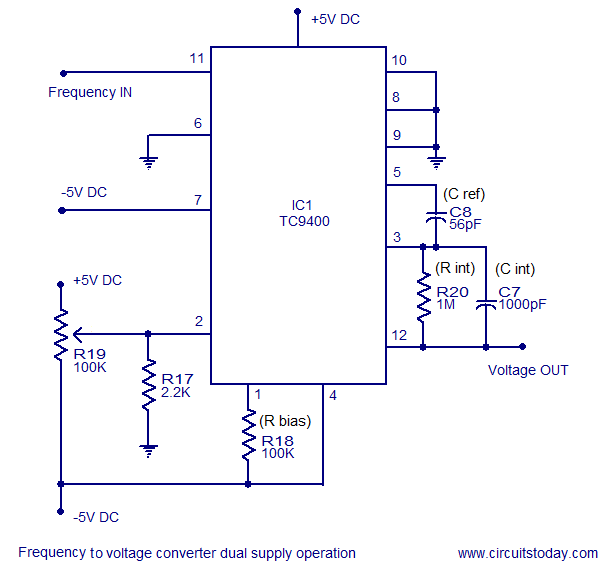Frequency to Voltage converter (Dual supply Version)

TC9400 based frequency to voltage converter working from a dual supply is shown above . Compared to the single supply version, this circuit requires less number of components. This circuit can be powered using a +/-5V DC dual supply. The circuit works only on a bipolar input frequency and if the frequency available to you is unipolar (a positive pulse train), then convert it to bipolar using the circuit shown below.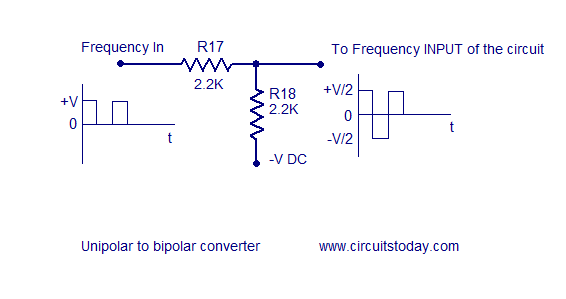Unipolar to Bipolar wave converter circuit

### Ripple eliminator circuit.

The output voltage of the 9400 based F to V converter is super imposed with a saw tooth ripple voltage which is inversely proportional to the value of the integrating capacitor Cint. For low frequency inputs the ripple voltage can be reduced by increasing the value of Cint. But this method is not suitable for high frequency inputs because the larger capacitance value will dramatically reduce the response time of the converter circuit. A method for reducing the output ripple voltage without affecting the circuits response time is shown below.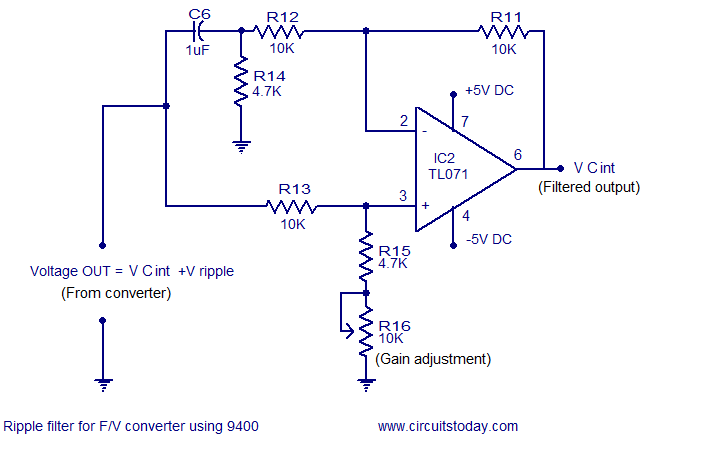Ripple eliminator circuit

The circuit is nothing but an operational amplifier working in the common mode. Due to the common mode operation the AC ripple is rejected and pure DC level will be available at the output. Trimpot R16 is used to adjust gain of inverting and non inverting inputs to the same value. This circuit will also act as a buffer.

### Power supply circuits for this project.

The power supply circuits required for the frequency to voltage converter project are shown below. The 12V DC supply can be used for powering the single supply version while the +/-5 V DC dual supply can be used for powering the dual supply version as well as the ripple filter circuit. In the circuit diagrams bridges D4 and D3 can be made using 1N4007 diodes. 1A bridge rectifier modules are also available in the market.You can follow what ever which is more convenient. Optional ON/OFF switches can be added in series to the phase lines of the AC input.It is advised to fit adequate heat sink ( 2x2x2 cm finned Aluminium or anything like that) to the voltage regulator ICs.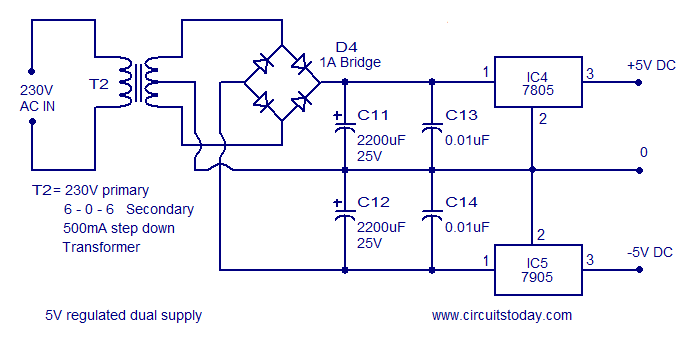5V dual regulated power supply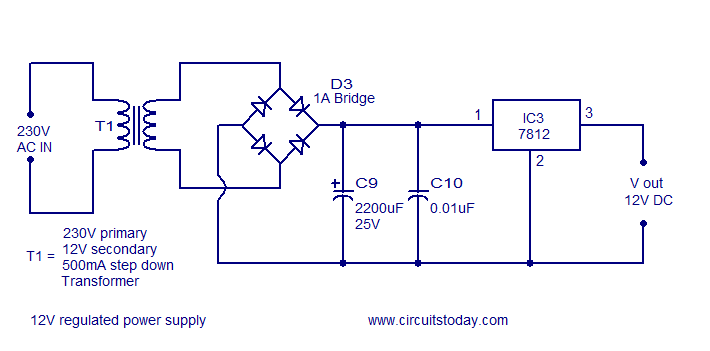12V regulated power supply

### Few other frequency to voltage converter circuits.

1.Frequency to voltage converter using Lm331: A very compact and preciseVoltage to Frequency converter using the renowned Lm331 IC from National Semiconductors. The circuit is highly linear and has a very great dynamic range.The circuit operated from a single supply and can be easily assembled on a Perf board.

2.Frequency to voltage converter using NE555: This is one great application of NE555 timer IC. A very simple and cheap F to V converter tha operates from 12VDC. Uses minimum number of components and almost all components required for this circuit can be obtained from your scrap box. Is it nice????. Have a try.

[Lights][grids]